# Flip-flop types, their Conversion and Applications

• Difficulty Level : Easy
• Last Updated : 06 Jun, 2022

Flip-flop is a circuit that maintains a state until directed by input to change the state. A basic flip-flop can be constructed using four-NAND or four-NOR gates. Types of flip-flops:

1. RS Flip Flop
2. JK Flip Flop
3. D Flip Flop
4. T Flip Flop

Logic diagrams and truth tables of the different types of flip-flops are as follows:

### S-R Flip Flop :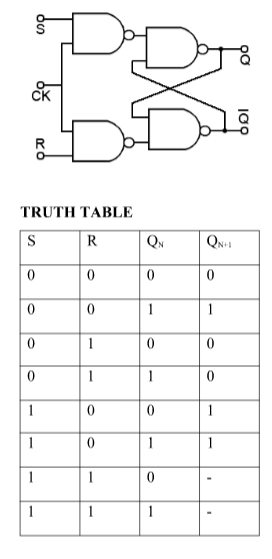Characteristics Equation for SR Flip Flop: QN+1 =  QNR’ + SR’

### J-K Flip Flop: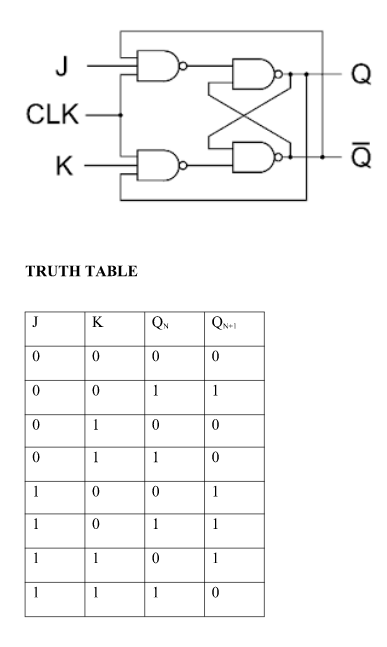Characteristics Equation for JK Flip Flop: QN+1 = JQ’N + K’QN

### D Flip Flop:

Characteristics Equation for D Flip Flop: QN+1 = D

### T Flip Flop:

Characteristics Equation for T Flip Flop: QN+1 = Q’NT + QNT’ = QN XOR T

### Conversion for Flip Flops:

EXCITATION TABLE: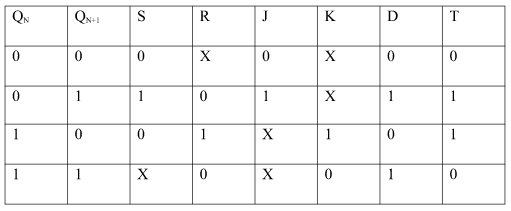Steps To Convert from One Flip Flop to Other :

Let there be required flipflop to be constructed using sub-flipflop:

1. Draw the truth table of the required flip-flop.
2. Write the corresponding outputs of sub-flipflop to be used from the excitation table.
3. Draw K-Maps using required flipflop inputs and obtain excitation functions for sub-flipflop inputs.
4. Construct a logic diagram according to the functions obtained.

i) Convert SR To JK Flip Flop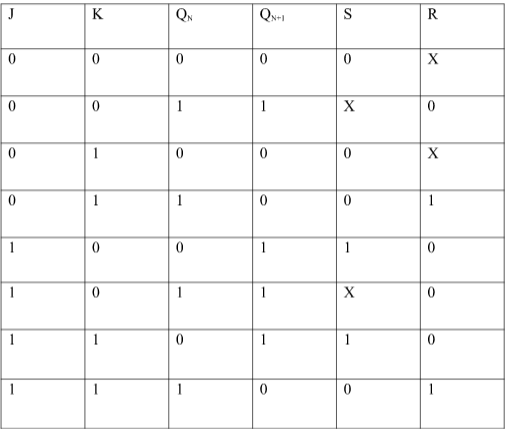Excitation Functions:

ii) Convert SR To D FlipFlop: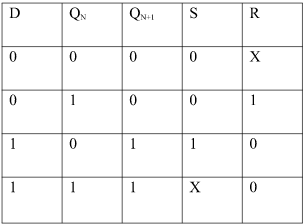Excitation Functions: S = D, R = D

###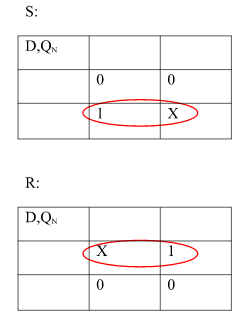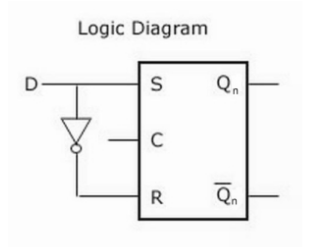Applications of Flip-Flops:

These are the various types of flip-flops being used in digital electronic circuits and the applications of Flip-flops are as specified below.

• Counters
• Frequency Dividers
• Shift Registers
• Storage Registers
• Bounce elimination switch
• Data storage
• Data transfer
• Latch
• Registers
• Memory